## Magnetic Field Around Solenoid

Magnetic Field around a Solenoid

Picture given below shows the solenoid. A typical solenoid behaves like a bar magnet. Magnetic field produced by solenoid is constant inside the solenoid and parallel to the axis of it.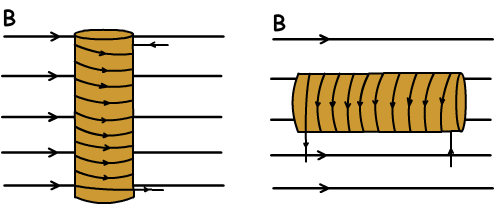We find the magnetic field produced by solenoid with the following formula;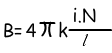Where: i is the current, N is the number of loops and l is the length of the solenoid.

We find the direction of magnetic field by using right hand rule again. Grab the solenoid as your four fingers shows the direction of current and your thumb shows the direction of magnetic field.

Example: Find the magnetic field produced by the solenoid if the number of loop is 400 and current passing through on it is 5 A.( Length of the solenoid is 40cm and k=10-7N/Amps2)

N=400, i=5A, l=40cm=0.4m, k=10-7N/Amps2,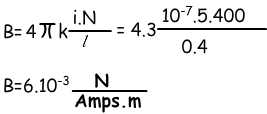Example: A solenoid has 80 cm diameter, number of loop is 4 and magnetic field inside it is 1,2 .10-5N/Amp.m. Find the current passing through the each loop of wire.

Since the questions ask current on each loop, we assume each loop as circle thus we find the magnetic field;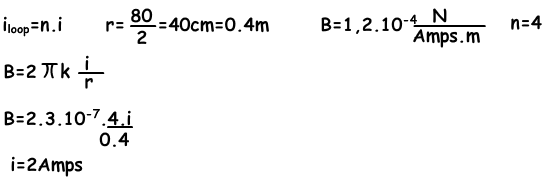Example: There are two solenoid given below, they have equal lengths and i1=4Amps and i2=3Amps.  Find the magnetic field vector at point A.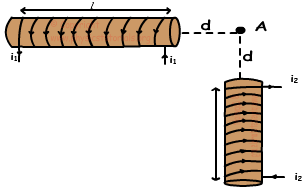i1 current produces B1 magnetic field and i2 current produces B2 magnetic field. We sum these vectors using vector properties and get following total magnetic field vector at point A.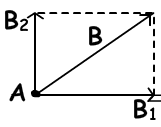Magnetism Exams and Solutions

Author:

Tags: